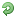# Thread: Manually calculating date difference

1. ##Manually calculating date difference

I need to teach my child to calculate the difference of two dates (same year and different years), what is the easiest method that an 8 year old child can follow?

TIAReply With Quote

2. ## Re: Manually calculating date difference

1) Write down number of days from start date until end of that month.
2) Write down number of days in each remaining month until the end of that year
3) Write down number of days in each remaining year until end year
4) Write down number of days in each month in end year up to end month
5) Write down number of days in end month until end dateReply With Quote

3. ## Re: Manually calculating date difference

We were taught this method in an insurance salesman training class, for calculating the age of an applicant based on the date of birth and the date on which he takes the policy. Let's assume your date of birth is 13 April 1989 and you want to calculate the age till today, which is 23 Jan 2011 here.

Write down both dates below each other (the larger one being on top) in dd mm yyyy format:

Code:
```  23 01 2011
- 13 04 1989
------------```
First subtract the dd from the dd. Your equation would now look like this:

Code:
```  23 01 2011
- 13 04 1989
------------
10```
Now subtract mm from mm. Here the number to be subtracted is greater, so 'borrow' one from the right hand side. Here's the trick: the right side represents a year. So if you borrow one from the year, you have to add 12 to the mm figure. After 'borrowing', the mm value on top becomes 12+1 = 13. So now the calculation looks like:

Code:
```  23 01(+12) 2011(-1)
- 13 04      1989
------------
10 09```
Now, do the same for the years. Here you have already 'borrowed' a year from the original figure, so reduce it from the year on top and then subtract the second year from it. So the final result looks like:

Code:
```  23 01(+12) 2011(-1)
- 13 04      1989
---------------------
10 09        21```
So the age would be 21 years, 09 months and 10 days.

This won't give you precisely the number of days between two dates, but it's handy enough for most needs. The precision will be affected due to leap years and months having different days. The calculation assumes each month has 30 days, for the sake of simplicity.

If you were to subtract a date like 26 April 1989, for e.g. you would have to do the borrowing operation at the month level as well. So it would look like:

Code:
```  23 01 2011
- 26 04 1989
------------

23(+30) 01(-1) 2011
- 26      04     1989
---------------------
27

23(+30) 01(-1+12) 2011(-1)
- 26      04        1989
----------------------------
27      08

23(+30) 01(-1+12) 2011(-1)
- 26      04        1989
----------------------------
27      08          21```
Good enough for an 8-year old, I guess.

.Reply With Quote

4. ## Re: Manually calculating date difference

You can correct HoneyBee's method at the cost of a table lookup, a single digit addition/subtraction, a low-digit multiplication and addition, and figuring out how many leap years your dates cross. I dunno whether or not an 8 year old would be able to remember it all, though it's computationally easier than zaza's.

To be more explicit, you can keep a table of how far off each month is from the case where every month has 30 days. You can keep a cumulative version which is more convenient for computation. For instance, "January = 1" since January has 31 days instead of 30. Then, "February = -1" since February has 28 days instead of 30, making us subtract 2 from January's 1. [Leap year is included at the end.] With a little more effort you can use this list to calculate the number of days between two months, then you can figure in the days on those months, then years, then leap years. The overall step-by-step algorithm should be relatively brief, at least.Reply With Quote

5. ## Re: Manually calculating date difference

You could do it this way, too:

1) Create/print a table that shows the day of year for each calendar date.

2) Create/print another table that shows how many days each year is from 1960 (or whatever year you choose).

Then all your daughter has to do is:

1) Find out how many days year 1 is from year 2,

2) Subtract the day-of-year of date 1 from that number.

3) Add the day-of-year of date 2 to that number.

Note: You may wish to write the instructions down so your daughter can refer to them.Reply With Quote

6. ## Re: Manually calculating date difference

I made a visual example on how to find the number of days in each month.

Hold your left hand as shown in figure. Then, start from the second finger, ie. January is the second finger, 3rd finger is for February, and so on.

Those humpy ones (2nd finger, 4th finger, 5th finger) are those months with 31 days. The rest will be having 30 days (except February) only.If you can't see the image, try this link : http://img718.imageshack.us/i/mathshand.png/Reply With Quote

####Posting Permissions

• You may not post new threads
• You may not post replies
• You may not post attachments
• You may not edit your posts
•

Featured